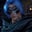Related Tags

binary tree
communitycreator

# How to do a post-order traversal of a binary tree using stacksGutha Vamsi Krishna

Post-Order traversal is a way of traversing a binary tree and follows the pattern below:

1. Visit the left part of the node.
2. Visit the right part of the node.
3. Visit the node.

In this shot, we use two stacks to traverse the binary tree in post-order.

### Algorithm

• Initialize two stacks and an array.

• Check if the root element is null. If it is null, then the tree is empty and returns an empty array.

• Otherwise, push the root element to stack 1.

• While stack 1 is not empty:

1. Remove the top element from stack 1.
2. Push that element to stack 2.
3. Check if the stack has left and right elements present. If present, push them onto stack 1, first pushing the left child and then the right child.
• If stack 1 becomes empty, the while loop will end.

• Now pop all elements from stack 2 and add them into the array.

• The array list will contain the elements traversed in a post-order fashion.

• Return the array.

Initialize two empty stacks
1 of 17

### Code

import java.util.*;

class Solution {
public List<Integer> postorderTraversal(TreeNode root) {

//initializes two stacks and one array list
Stack<TreeNode> st1 = new Stack<>();
Stack<TreeNode> st2 = new Stack<>();
List<Integer> postorder = new ArrayList<>();

//checks if tree is empty
if(root == null) return postorder;

//push root node into stack 1
st1.push(root);

//loop executes till stack 1 is not empty
while(!st1.isEmpty()){

//remove top element from stack 1
root = st1.pop();

//push removed element to stack 2
st2.push(root);

//check if it has any child, if present then push into stack 1
if(root.left != null) st1.push(root.left);
if(root.right != null) st1.push(root.right);
}

//add elements from stack 2 to array list
while(!st2.isEmpty()){
}

//return the postorder list
return postorder;
}
}

//class to create a new node in tree
class TreeNode {
int val;
TreeNode left;
TreeNode right;
TreeNode() {}
TreeNode(int val) { this.val = val; }
TreeNode(int val, TreeNode left, TreeNode right) {
this.val = val;
this.left = left;
this.right = right;
}
}

class Main{
public static void main(String[] args){

//create instances of nodes
TreeNode node1 = new TreeNode(1);
TreeNode node2 = new TreeNode(2);
TreeNode node3 = new TreeNode(3);
TreeNode node4 = new TreeNode(4);
TreeNode node5 = new TreeNode(5);
TreeNode node6 = new TreeNode(6);
TreeNode node7 = new TreeNode(7);
TreeNode node8 = new TreeNode(8);

//creating a binary tree as shown in above represention
node1.left = node2;
node1.right = node3;

node2.left = node4;
node2.right = node5;

node3.right = node6;

node6.right = node7;

node7.right = node8;

//constructing solution object
Solution solution = new Solution();

//printing out the returned array list from solution
System.out.println(solution.postorderTraversal(node1));
}
}

RELATED TAGS

binary tree
communitycreator

CONTRIBUTORGutha Vamsi Krishna
RELATED COURSES

View all Courses

Keep Exploring

Learn in-demand tech skills in half the time